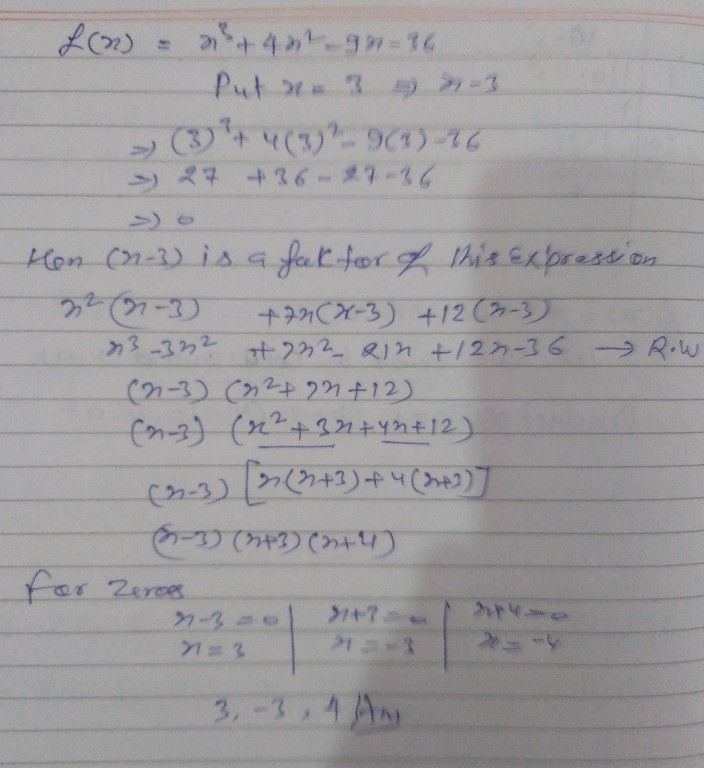Symbol
ProblemPolynomials EXAMPLE $5.$ Find the zeroes of the polynomial $f\left(x\right)=x^{3}+4x^{2}-9x-36$ $ftw$ two o zeroes are equal in magnitude but opposite in sign.